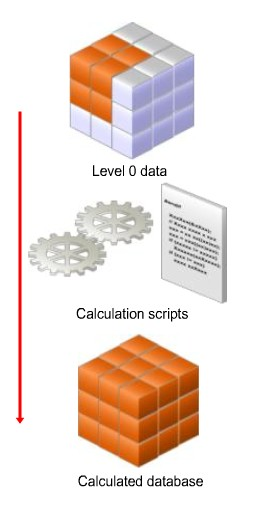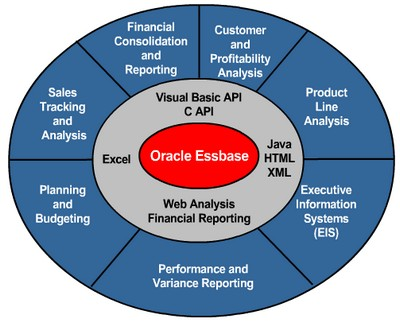# Essbase - Outline-based Calculations

Outline Calculations represent the simplest method of calculations. In block storage outline calculations, Essbase calculates the essbase database according to :

During a batch calculation process, you initiate outline calculations by executing the default database calculation. The default calculation contain the calculation script command CALC ALL. This calculation command calculates and aggregate the entire database, based on the consolidation operator and formulas in the database outline.

## Article Related

Discover MoreEssbase - Calculation Script

Calculation scripts are text files that define how to calculate data in the database. In a block storage database, they represent the second method of calculations after the basic outline calculations...Essbase - Calculations

Essbase includes powerful calculation features for demanding analytic requirements. A rich library of functions makes it easy to define advanced and sophisticated business logic and relationships. Essbase...